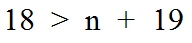Worksheets and No Prep Teaching Resources
Math Worksheets

Inequalities Worksheets

Understanding equalities becomes easier with lots of good practice, and these thorough, engaging worksheets will help students do just that. From equations to graphs, students can systematically work their way through this exciting topic. Problems include graphing, solving linear equations, multi-step inequalities, and absolute value problems for a complete unit on this important math topic.Inequalities
Determine the truth of an inequality
Solving inequalities by adding or subtracting (whole numbers)
Solving inequalities by adding or subtracting (fractions)
Solving Inequalities by multiplying or dividing
Solving Multi-Step Inequalities
Inequalities Word Problems Printable
Inequalities Review Printable

Algebra Word Problems and Critical Thinking Problems
Inequalities Problems

Graphing Inequalities
Graphs of Inequalities - Draw the graph
Graphs of Inequalities - Draw the graph (Includes Graph Paper)
Solving Inequalities

Linear Equations
Inequalities - Sketch the graph of the inequality
Inequalities and Absolute Value - Sketch the graph

Systems of Linear Equations and Inequalities
Linear Systems - Solve by graphing
Linear Systems - Solve by graphing (graph paper on worksheet)
Linear Systems - Solve by using the substitution method (in Ax + By = C format)
Linear Systems - Solve by using the substitution method
Linear Systems - Solve by using the elimination method (in Ax + By = C format)
Linear Systems - Solve by using the elimination method
Linear Systems - Solve by using any method (in Ax + By = C format)
Linear Systems - Solve by using any method
Inequalities - Solve each system of inequalities by graphing (3 vertices)
Inequalities - Solve each system of inequalities by graphing (4 vertices)
Inequalities - Solve each system of inequalities by graphing (3-4 vertices)
Linear Programming - Find the minimum and maximum (3 vertices)
Linear Programming - Find the minimum and maximum (4 vertices)
Linear Programming - Find the minimum and maximum (3-4 vertices)
Systems of Linear Equations in Three Variables - Solve (easier problems)
Systems of Linear Equations in Three Variables - Solve
Review

Have a suggestion or would like to leave feedback?